# Mr. Heltenberg

•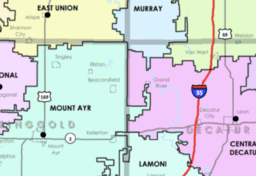### School Districts (what school is closer)

Activity

Mr. Heltenberg

•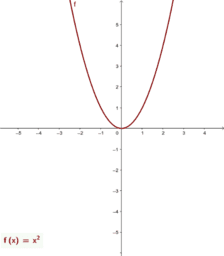### The end behavior of a polynomial function Mr. Heltenberg

Activity

Mr. Heltenberg

•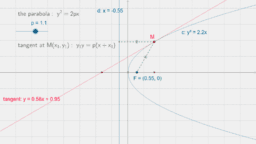### Mr. Heltenberg parabola (equation)

Activity

Mr. Heltenberg

•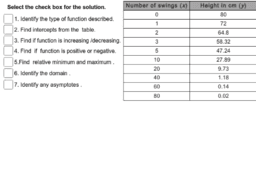### Identify the key features of the function (Heltenberg)

Activity

Mr. Heltenberg

•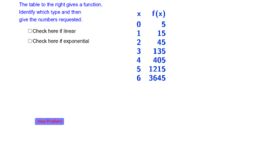### Exponential vs Linear from Table Mr. Heltenberg

Activity

Mr. Heltenberg

•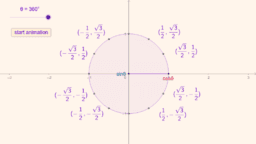### basic trig Unit Circle

Activity

Mr. Heltenberg

•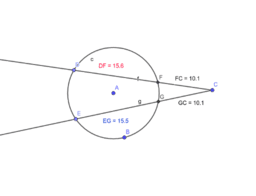### Segments of Chords Theorem (point outside)

Activity

Mr. Heltenberg

•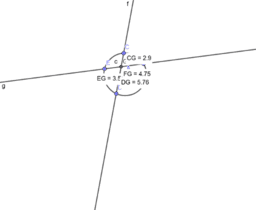### Segments of Chords Theorem (point inside)

Activity

Mr. Heltenberg

•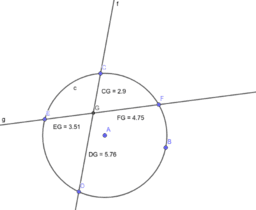### Segments of Chords Theorem

Activity

Mr. Heltenberg

•### Limit and First Principle

Activity

Mr. Heltenberg

•### Square

Activity

Mr. Heltenberg